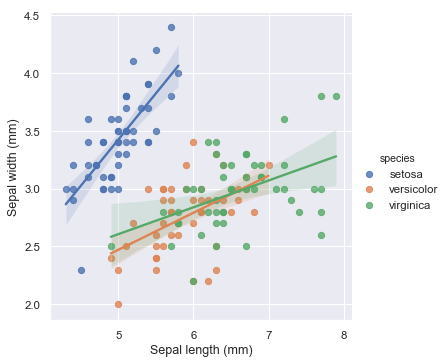Categories

## Multiple Linear Regression

In Multiple Linear Regression, we try to find the relationship between 2 or more independent variables (inputs) and the corresponding dependent variable (output). The independent variables can be continuous or categorical.

The equation that describes how the predicted values of y is related to p independent variables is called as Multiple Linear Regression equation :

Below is the graph for Multiple Linear Regression Model, applied on the iris data set:Graph for Multiple Linear Regression Model

# Multiple linear regression analysis can help us in the following ways :

It helps us predict trends and future values. The multiple linear regression analysis can be used to get point estimates.

It can be used to forecast the effects or impacts of changes. That is, multiple linear regression analysis can help to understand how much will the dependent variable change when we change the independent variables.

It can be used to identify the strength of the effect that the independent variables have on a dependent variable.

Categories

## Simple

In Simple Linear Regression, we try to find the relationship between a single independent variable (input) and a corresponding dependent variable (output). This can be expressed in the form of a straight line.

The same equation of a line can be re-written as:

Y represents the output or dependent variable.

β0 and β1 are two unknown constants that represent the intercept and coefficient (slope) respectively.

ε (Epsilon) is the error term.

The following is a sample graph of a Simple Linear Regression Model :

# Applications of Simple Linear Regression include :

1) Predicting crop yields based on the amount of rainfall: Yield is dependent variable while the amount of rainfall is independent variable.

2) Marks scored by student based on number of hours studied (ideally) : Here marks scored is dependent and number of hours studied is independent.

3) Predicting the Salary of a person based on years of experience : Thus Experience become the independent variable while Salary becomes the dependent variable.

Categories

# Linear Regression is generally classified into two types:

1) Simple Linear Regression

2) Multiple Linear Regression